Data

# Technical Tuesday: Using Snowflake’s Generator Function to Create Date and Time Scaffold Tables

Of the many useful functions available in Snowflake, one of my favourites is the GENERATOR function. This function allows you to create rows of data from nothing and can be useful in many ways. Any time you don’t have physical data to get you started but you know how you want to create it, I would recommend considering the GENERATOR function as a way to get you there. With this function, I have created all sorts of basic dummy data objects on which to test functions and code without touching “real” data, including the dummy data of quantities per category in the first example below.

For production-level object examples instead of simple dummy data sets, we have date and time scaffold tables. Scaffolding is often required when transforming data to ensure a record exists for each occurrence of a given timeframe, such as weeks, days, hours, minutes, etc. I have used the code contained below to create date and time scaffolds for several clients for various reasons, such as populating records between the “CreateDate” and “CloseDate” of a data point. Scaffolding your data can be the key to creating analyses such as the current number of open tickets on a given day or displaying the number of active events at a given time.

## A Simple Example

Before we cover any specifically useful examples, let’s first cover the basics of the GENERATOR function. The function accepts two optional parameters:

• rowcount – The number of rows that the function will generate
• timelimit – The number of seconds for which the function will generate records

If neither parameter is provided, the function will simply return no records. If both are provided, the function will return records based on whichever parameter is reached first.

The GENERATOR function is always paired with the TABLE function to produce a result that can be queried. We can see this in our first example now, for which we will simply output the same value five times.

```SELECT
'MY_VALUE' as "MY_FIELD_NAME"
FROM TABLE(GENERATOR(rowcount => 5) )```

If we execute this code in Snowflake, it will yield the following result: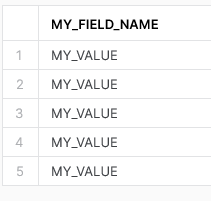As you can see, our output contains five records where each value matches our given hard-coded input.

## A Slightly More Complex Example

Now that we have covered a basic example, let’s demonstrate something a bit more useful. We can use GENERATE to create tables with any sequence or function that does not require an input. This includes functions such as ROW_NUMBER and data generation functions such as SEQ4.

For this example, we will simply combine a few of these to demonstrate the functionality:

```SELECT
seq4() -- sequence of 4-byte integers
, random() -- pseudo-random 64-bit integer.
, ROW_NUMBER() OVER (ORDER BY seq4()) as "ROW_NUMBER" -- window function to determine the row number, in the order of the
FROM TABLE(GENERATOR(rowcount => 5) )```

This yields the following result: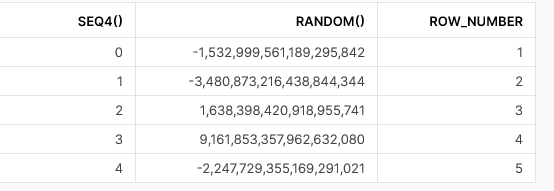Whilst this is nothing meaningful or significant on its own, it builds as strong foundation for the more useful example below, and the date and time scaffold tables at the end of this blog post.

Two things I would like to highlight at this stage:

1. SEQ4 does not necessarily produce what is known as a gap-free sequence. When using functions such as SEQ4, it is possible for the output to be missing values in the sequence depending on the logic that you are applying. To avoid this risk, we can use ROW_NUMBER instead. ROW_NUMBER will not leave gaps because it is calculated based on the window of the output after any other logic may have taken place.
2. ROW_NUMBER starts from 1 whilst SEQ4 starts from 0. When we generate values using ROW_NUMBER later in this post, we deduct 1 so that our ROW_NUMBER values also start from 0. This ensures that our first record matches our original input instead of immediately incrementing; for example, if we have a specific start date in mind for our calendar table

## A More Useful Example

I often find myself looking to create quick demonstrations of Snowflake functionality or mock up a potential solution, and GENERATOR allows me to quickly create a dummy dataset to demonstrate a concept.

Perhaps I wish to create a dummy dataset of quantities across three categories. With GENERATOR, I can create a table with a predefined number of records and leverage the UNIFORM and RANDOM functions to created randomised values between given ranges for each record.

We can see this in action here with the below script. As you can see, there is not a lot to unpack here when it comes to the GENERATOR itself, which is only present in the final line. Most of the complexity in this script is from the UNIFORM and RANDOM functions.

```SELECT
CASE UNIFORM(1, 3, RANDOM() )
WHEN 1 THEN 'CATEGORY_A'
WHEN 2 THEN 'CATEGORY_B'
WHEN 3 THEN 'CATEGORY_C'
END AS "CATEGORY"
, UNIFORM(1, 500, RANDOM() ) as "QUANTITY"
FROM TABLE(GENERATOR(rowcount => 100) )```

This script achieves the simple result of creating a table with 100 records, populating each value with the results of our UNIFORM and RANDOM combination. This yields a simple yet effective result: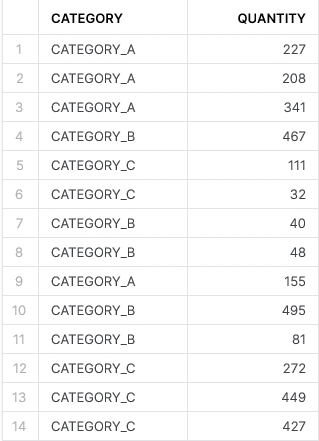To achieve this result, the key components have been the pairing of TABLE and GENERATOR to create a table with the desired number of records and the pairing of UNIFORM and RANDOM to populate the field values.

NOTE: Every time the code above is executed, new values will be received from the RANDOM function. If you wish to maintain the same values each time, you can simply enter a seed value as the parameter of the RANDOM function.

## Creating a Date Scaffold Table

Now that we have covered our basic GENERATOR example, we can move on to the date scaffold table. This is a more involved example but the GENERATOR component itself is tiny.

For this example, we want to create a table that contains a record for every date from the start of 1970 to the end of 2099. To achieve this, we use GENERATOR to create a table with enough rows to cover our timeframe, then convert the row number into a date. Once we have our dates, it is a simple matter of extract the relevant information from the date to create our full date scaffold table.

Note that we leverage ROW_NUMBER instead of simply calling a sequence. This is to ensure we do not have any gaps in our sequence, as this would result in missing dates in our output.

```CREATE OR REPLACE TABLE "DIM_DATE"
AS
-- Leverage ROW_NUMBER to ensure a gap-free sequence.
-- This is a CTE to allow "ROW_NUMBER" to be leveraged in window functions.
WITH "GAPLESS_ROW_NUMBERS" AS (
SELECT
ROW_NUMBER() OVER (ORDER BY seq4()) - 1 as "ROW_NUMBER"
FROM TABLE(GENERATOR(rowcount => 366 * (2100 - 1970)) ) -- rowcount is 366 days x (2100 - 1970) years to cover leap years. A later filter can remove the spillover days
)
SELECT
DATEADD('day', "ROW_NUMBER", DATE(0)) as "DATE" -- Dimension starts on 1970-01-01 but a different value can be entered if desired
, EXTRACT(year FROM "DATE") as "YEAR"
, EXTRACT(month FROM "DATE") as "MONTH"
, EXTRACT(day FROM "DATE") as "DAY"
, EXTRACT(dayofweek FROM "DATE") as "DAY_OF_WEEK"
, EXTRACT(dayofyear FROM "DATE") as "DAY_OF_YEAR"
, EXTRACT(quarter FROM "DATE") as "QUARTER"
, MIN("DAY_OF_YEAR") OVER (PARTITION BY "YEAR", "QUARTER") as "QUARTER_START_DAY_OF_YEAR"
, "DAY_OF_YEAR" - "QUARTER_START_DAY_OF_YEAR" + 1 as "DAY_OF_QUARTER"
, TO_VARCHAR("DATE", 'MMMM') as "MONTH_NAME"
, TO_VARCHAR("DATE", 'MON') as "MONTH_NAME_SHORT"
, CASE "DAY_OF_WEEK"
WHEN 0 THEN 'Sunday'
WHEN 1 THEN 'Monday'
WHEN 2 THEN 'Tuesday'
WHEN 3 THEN 'Wednesday'
WHEN 4 THEN 'Thursday'
WHEN 5 THEN 'Friday'
WHEN 6 THEN 'Saturday'
END as "DAY_NAME"
, TO_VARCHAR("DATE", 'DY') as "DAY_NAME_SHORT"
, EXTRACT(yearofweekiso FROM "DATE") as "ISO_YEAR"
, EXTRACT(weekiso FROM "DATE") as "ISO_WEEK"
, CASE
WHEN "ISO_WEEK" <= 13 THEN 1
WHEN "ISO_WEEK" <= 26 THEN 2
WHEN "ISO_WEEK" <= 39 THEN 3
ELSE 4
END as "ISO_QUARTER"
, EXTRACT(dayofweekiso FROM "DATE") as "ISO_DAY_OF_WEEK"
, MAX("DAY_OF_YEAR") OVER (PARTITION BY "YEAR") as "DAYS_IN_YEAR"
, "DAYS_IN_YEAR" - "DAY_OF_YEAR" as "DAYS_REMAINING_IN_YEAR"
FROM "GAPLESS_ROW_NUMBERS"
WHERE "YEAR" < 2100 -- WHERE clause then restricts back to desired timeframe since 366 days per year when generating row numbers is too many```

We can see an example output here: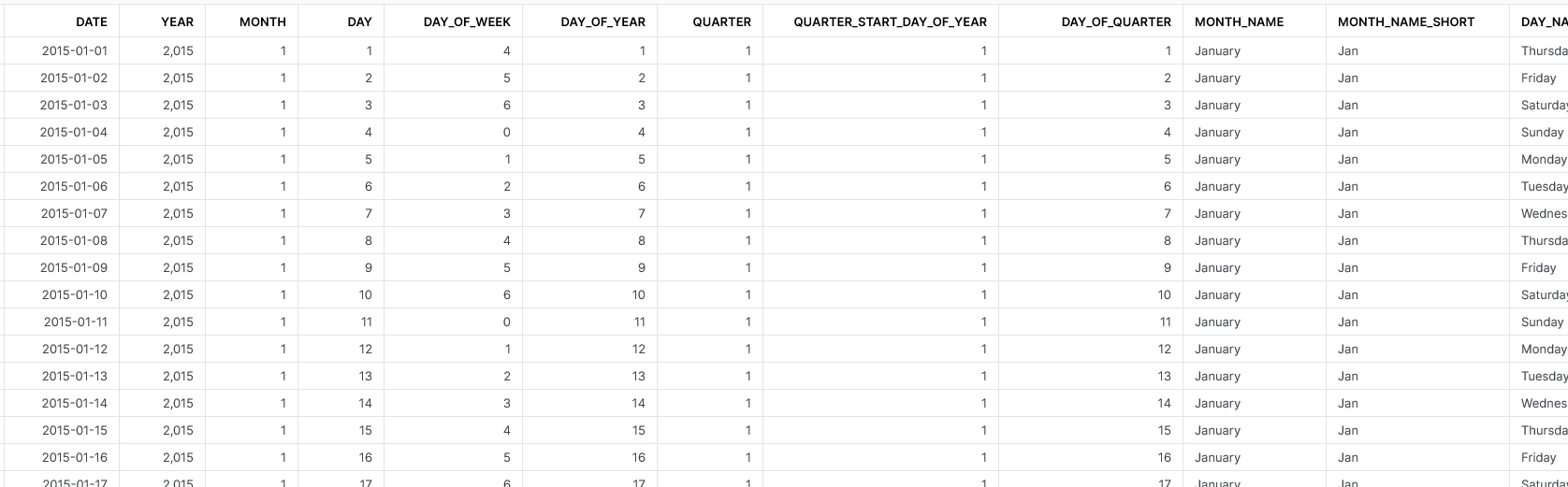## Creating a Time Scaffold Table

In a very similar fashion, we can also create a time scaffold table:

```CREATE OR REPLACE TABLE "DIM_TIME"
AS
-- Leverage ROW_NUMBER to ensure a gap-free sequence.
-- This is a CTE to allow "ROW_NUMBER" to be leveraged in window functions.
WITH "GAPLESS_ROW_NUMBERS" AS (
SELECT
ROW_NUMBER() OVER (ORDER BY seq4()) - 1 as "ROW_NUMBER"
FROM TABLE(GENERATOR(rowcount => 60*60*24) ) -- rowcount is 60s x 60m x 24h
)
SELECT
TIMEADD('second', "ROW_NUMBER", TIME('00:00')) as "TIME" -- Dimension starts at 00:00
, EXTRACT(hour FROM "TIME") as "HOUR"
, EXTRACT(minute FROM "TIME") as "MINUTE"
, EXTRACT(second FROM "TIME") as "SECOND"
, TO_VARCHAR("TIME", 'HH12:MI:SS AM') as "TIME_12H"
, "MINUTE" = 0 and "SECOND" = 0 as "HOUR_FLAG"
, "MINUTE"%15 = 0 and "SECOND" = 0 as "QUARTER_HOUR_FLAG"
, "HOUR" >= 6 and "HOUR" < 18 as "DAY_SHIFT_FLAG"
, NOT "DAY_SHIFT_FLAG" as "NIGHT_SHIFT_FLAG"
, IFF("HOUR" < 12, 'AM', 'PM') as "TIME_PERIOD"
FROM "GAPLESS_ROW_NUMBERS"```

We can see an example output here: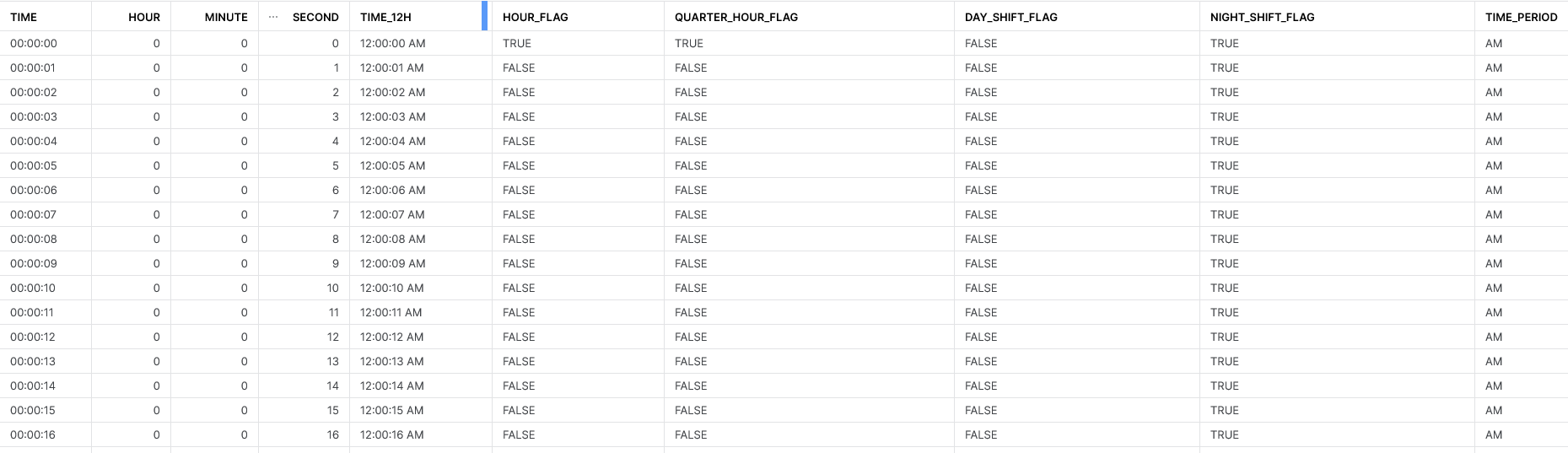I hope you find some of the code and explanations here to be useful. I’d be interested to know if you think of any interesting ways to leverage GENERATOR too, so please let me know what you come up with!

### The Latest

• ##### Tableau Relationships: Basics – The Ground Rules

A Definitive Guide to Python UDFs in the Snowflake UI Snowpark for Python is the name for the new Python functionality integration that Snowflake has recently developed. At the Snowflake ...
Technical Tuesday: Using Snowflake’s Generator Function to Create Date and Time Scaffold Tables Of the many useful functions available in Snowflake, one of my favourites is the GENERATOR function. This function allows you to create ...

See more from this author →

• I understand that InterWorks will use the data provided for the purpose of communication and the administration my request. InterWorks will never disclose or sell any personal data except where required to do so by law. Finally, I understand that future communications related topics and events may be sent from InterWorks, but I can opt-out at any time.
• This field is for validation purposes and should be left unchanged.

InterWorks uses cookies to allow us to better understand how the site is used. By continuing to use this site, you consent to this policy. Review Policy OK

×

Interworks GmbH
Ratinger Straße 9
40213 Düsseldorf
Germany
Geschäftsführer: Mel Stephenson

Kontaktaufnahme: markus@interworks.eu
Telefon: +49 (0)211 5408 5301

Amtsgericht Düsseldorf HRB 79752
UstldNr: DE 313 353 072